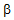# Electronic Devices - BJT Amplifiers

### Exercise :: BJT Amplifiers - General Questions

26.

Which of the following represent(s) the advantage(s) of the system approach over the r-model approach?

 A. Thevenin's theorem can be used. B. The effect of changing the load can be determined by a simple equation. C. There is no need to go back to the ac equivalent model and analyze the entire network. D. All of the above

Explanation:

No answer description available for this question. Let us discuss.

27.

The differential amplifier has

 A. one input and one output. B. two inputs and two outputs. C. two inputs and one output. D. one input and two outputs.

Explanation:

No answer description available for this question. Let us discuss.

28.

The emitter-follower configuration has a ________ impedance at the input and a ________ impedance at the output.

 A. low, low B. low, high C. high, low D. high, high

Explanation:

No answer description available for this question. Let us discuss.

29.

The differential amplifier produces outputs that are

 A. common mode. B. in-phase with the input voltages. C. the sum of the two input voltages. D. the difference of the two input voltages.

Explanation:

No answer description available for this question. Let us discuss.

30.

The ________ model suffers from being limited to a particular set of operating conditions if it is to be considered accurate.

 A. hybrid equivalent B. re C.D. Thevenin